# Class 7 Maths NCERT Solutions for Chapter – 3 Data Handling EX – 3.3

## Data Handling

Question 1.
Use the bar graph to answer the following questions.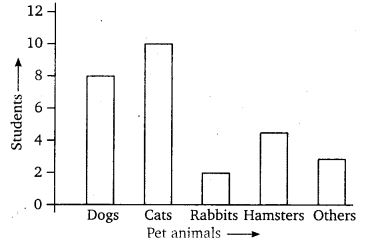(a) Which is the most popular pet?
(b) How many students have dog as a pet?

Solution:
Clearly, from the given bar graph :

(a) The most popular pet is cat.
(b) Eight children have dog as a pet.

Question 2.
Read the bar graph which shows the number of books sold by a bookstore during five consecutive years and answer the following questions :
(i)
About how many books were sold in 1989? 1990? 1992?
(ii) In which year were about 475 books sold? About 225 books sold?
(iii) In which years were fewer than 250 books sold?
(iv) Can you explain how you would estimate the number of books sold in 1989?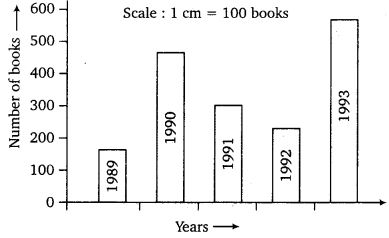Solution:
Clearly, from the given graph, we have
(i) Number of books sold in the year
1989 : 170 (approx.)
1990 : 475 (approx.)
1992 : 225 (approx.)
(ii) In the year 1990, about 475 books were sold. In the year 1992, about 225 books were sold.
(iii) Fewer than 250 books were sold in the years 1989 and 1992.
(iv) It can be estimated using the height of the bar such that height of 1 cm = 100 books.

Question 3.
Number of children in six different classes are given below. Represent the data on a bar graph.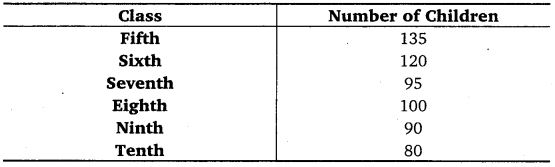(a) How would you choose a scale?
(b) Answer the following questions :
(i) Which class has the maximum number of children? And the minimum?
(ii) Find the ratio of students of class sixth to the student of class eighth.

Solution:
(a) Start the scale at 0. The greatest value in the data is 135, so end the scale at a value greater than 135, such as 140. Use equal divisions along the axes, such as increments of 10. Here, we take 1 unit for 10 children. The bar graph is as under :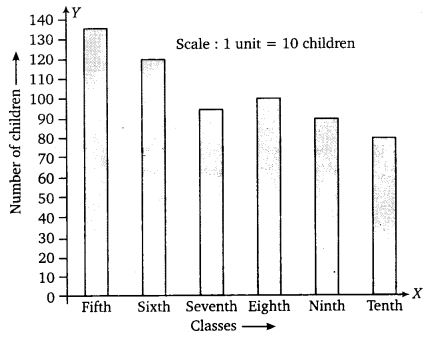(b) (i) The fifth class has the maximum number of children. The minimum number of children are in class tenth.
(ii) Ratio of students of class sixth to eighth is 120 : 100 = 6 : 5.

Question 4.
The performance of a student in 1st Term and 2nd Term is given. Draw a double bar graph choosing appropriate scale and answer the following :1. In which subject has the child improved his performance the most?
2. In which subject is the improvement the least?
3. Has the performance gone down in any subject?

Solution:
The graph is as under :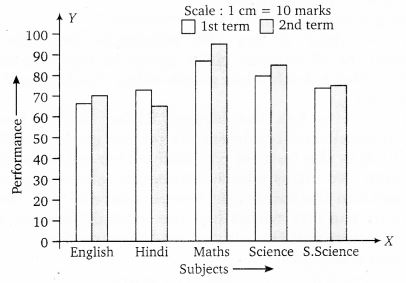1. In Mathematics the child has improved his performance the most.
2. In Social Science the child has improved his performance the least.
3. Yes, the performance has gone down in Hindi.

Question 5.
Consider this data collected from a survey of a colony.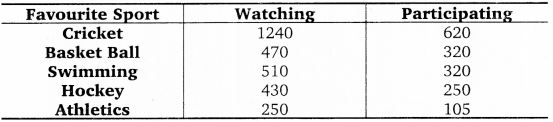(i) Draw a double bar graph choosing an appropriate scale. What do you infer from the bar graph?
(ii) Which sport is most popular?
(iii) Which is more preferred, watching or participating in sports?

Solution:
(i) The graph is as under :It is inferred that more people prefer cricket and less athletics.
(ii) Most popular sport is cricket.
(iii) Watching is more preferred than participating.

Question 6.
Take the data giving the minimum and the maximum temperature of various cities given in the beginning of this chapter. Plot a double bar graph using the data and answer the following :

1. Which city has the largest difference in the minimum and maximum temperature on the given date?
2. Which is the hottest city and which is the coldest city?
3. Name two cities where maximum temperature of one was less than the minimum temperature of the other.
4. Name the city which has the least difference between its minimum and the maximum temperature.

Solution:
The graph is as under :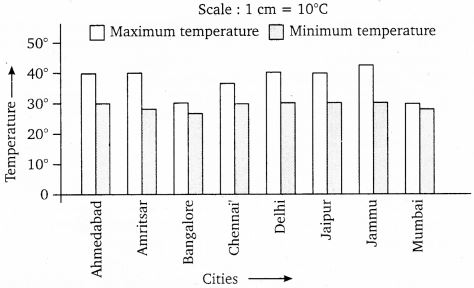1. The city Jammu has the largest difference in the minimum and maximum temperature on the given date.
2. Jammu is the hottest city and Bangalore is the coldest city.
3. The name of the two cities where maximum temperature of one was less than the minimum temperature of other are Bangalore and Jaipur or Bangalore and Ahmedabad.
4. Mumbai has the least difference between its minimum and the maximum temperature.